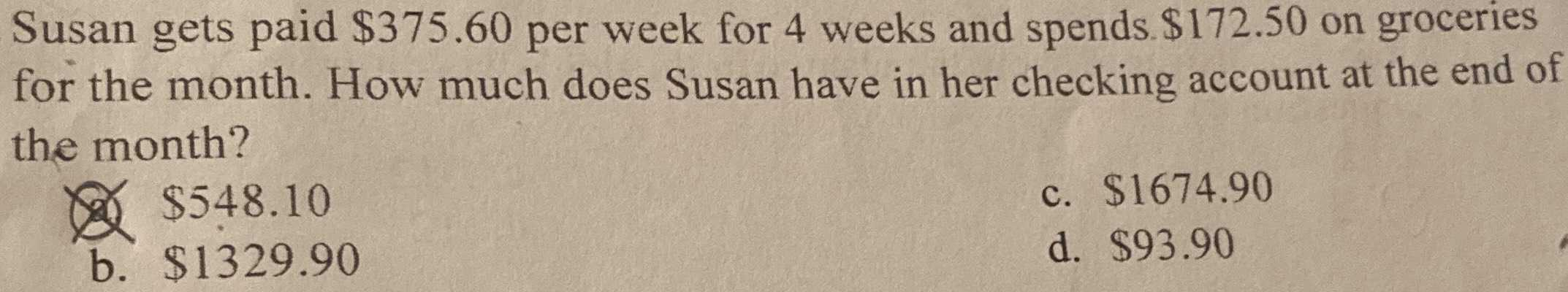### Still have math questions?

Arithmetic
QuestionSusan gets paid $$\ 375.60$$ per week for $$4$$ weeks and spends. $$\ 172.50$$ on groceries for the month. How much does Susan have in her checking account at the end of the month? 2 $$\ 548.10$$ c. $$\ 1674.90$$

b. $$\ 1329.90$$ d. $$\ 93.90$$